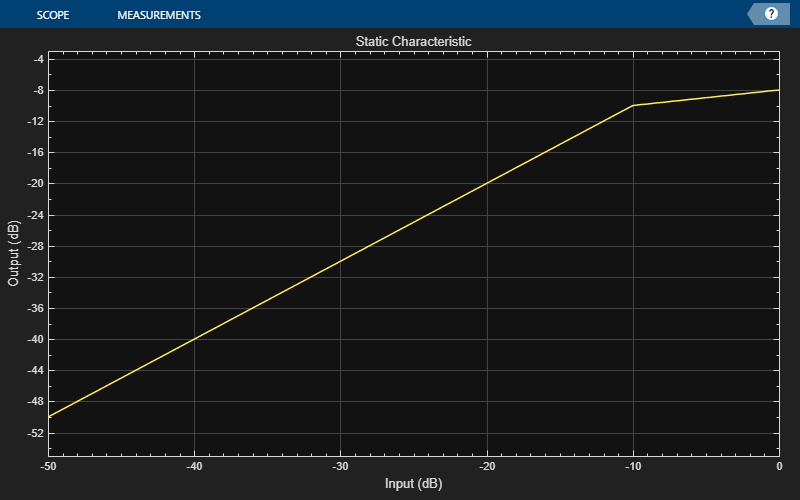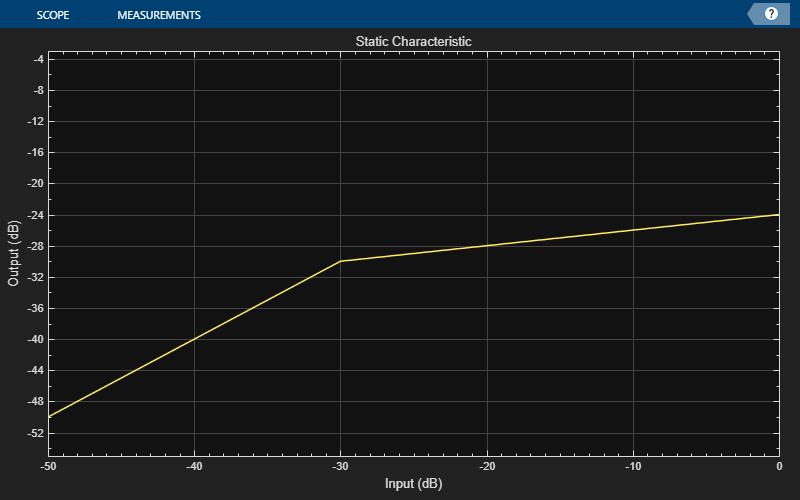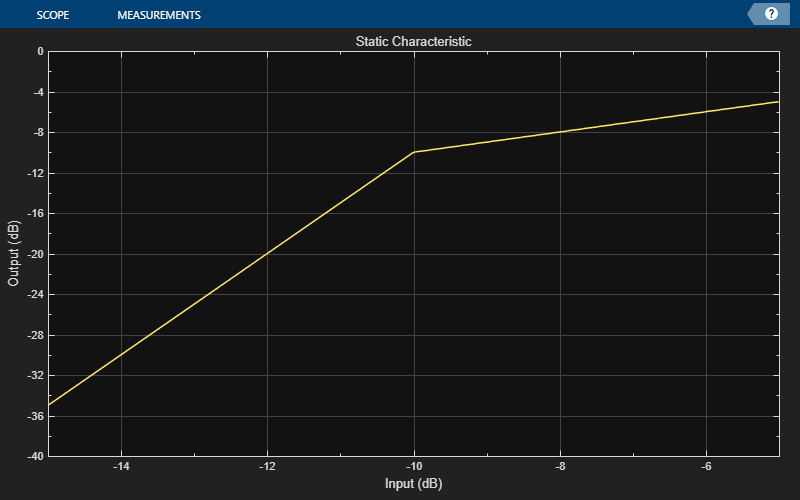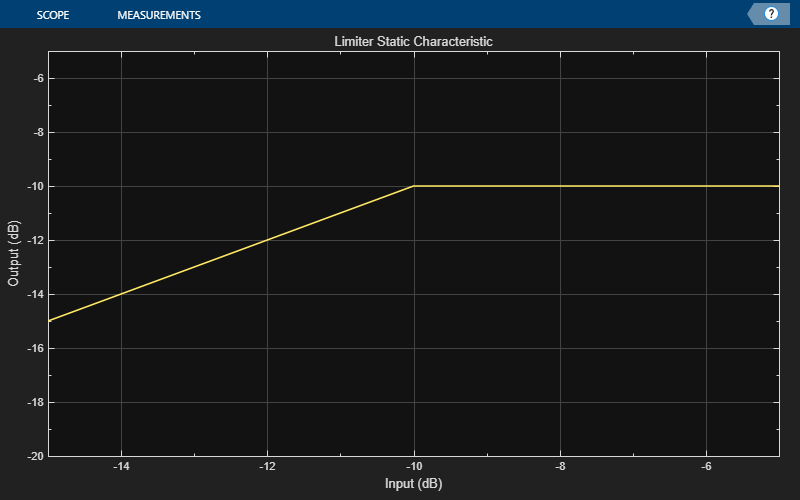# visualize

Visualize static characteristic of dynamic range controller

## Syntax

``visualize(dynamicRangeController)``
``visualize(dynamicRangeController,inputRange)``
``outputLevel = visualize(___)``

## Description

example

````visualize(dynamicRangeController)` plots the static characteristic of the dynamic range control object. The plot is updated automatically when properties of the object change.```

example

````visualize(dynamicRangeController,inputRange)` enables you to specify the input range.```

example

````outputLevel = visualize(___)` returns the dB output level corresponding to the input range. You can use any of the input arguments from previous syntaxes. NoteThis syntax is only available for the `compressor`, `limiter`, and `expander` System objects. It is not available for the `noiseGate` System object™. ```

## Examples

collapse all

Create an object of the `compressor` System object™, and then plot the static characteristic.

```dynamicRangeCompressor = compressor; visualize(dynamicRangeCompressor)```The static characteristic plot updates automatically if you modify a property of the object.

`dynamicRangeCompressor.Threshold = -30;`Create an object of the `expander` System object™. Plot the static characteristic over the range -15 to -5, in 0.001 dB increments.

```dynamicRangeExpander = expander; visualize(dynamicRangeExpander,-15:0.001:-5)```Create an object of the `limiter` System object™. Get the output level of the static characteristic over a specified range.

```dynamicRangeLimiter = limiter; inputLevel = -15:1:-5```
```inputLevel = 1×11 -15 -14 -13 -12 -11 -10 -9 -8 -7 -6 -5 ```
`outputLevel = visualize(dynamicRangeLimiter,inputLevel)`
```outputLevel = 1×11 -15 -14 -13 -12 -11 -10 -10 -10 -10 -10 -10 ```## Input Arguments

collapse all

Dynamic range control object, specified as an object of `compressor`, `expander`, `limiter` or `noiseGate`.

Range over which to calculate the output of the static characteristic.

The default input range depends on the dynamic range control object:

• `compressor` –– `[-50:0.01:0]` dB

• `limiter` –– `[-50:0.01:0]` dB

• `expander` –– `[-50:0.01:0]` dB

• `noiseGate` –– `[0:0.001:1]` linear

## Output Arguments

collapse all

Output level in dB, returned as a vector the same size as `inputRange`.

This output is only available for the `compressor`, `limiter`, and `expander` System objects. It is not available for the `noiseGate` System object.